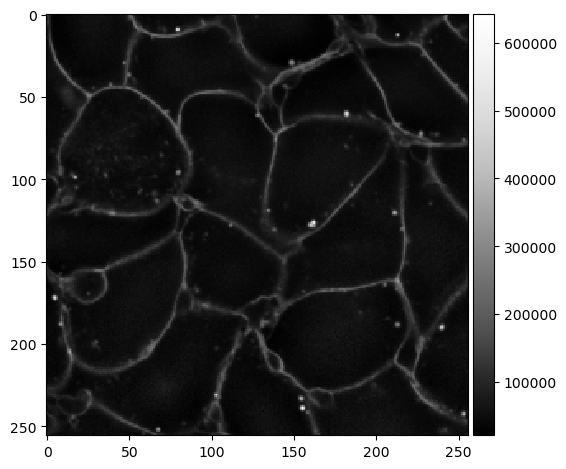# Divide by Gaussian#

When processing images with membrane staining it sometimes happens that the intensity of the membranes is not homogeneous and changes locally. This intensity variation may impair cell segmentation algorithms. In these cases it may make sense to homogenize the intensity by dividing the image by a Gaussian blurred version of itself.

import pyclesperanto_prototype as cle
from skimage.filters import gaussian


In this image you see that the intensity of the membranes decreases from top to bottom.

image = imread('../../data/membranes_2d.tif')
cle.asarray(image)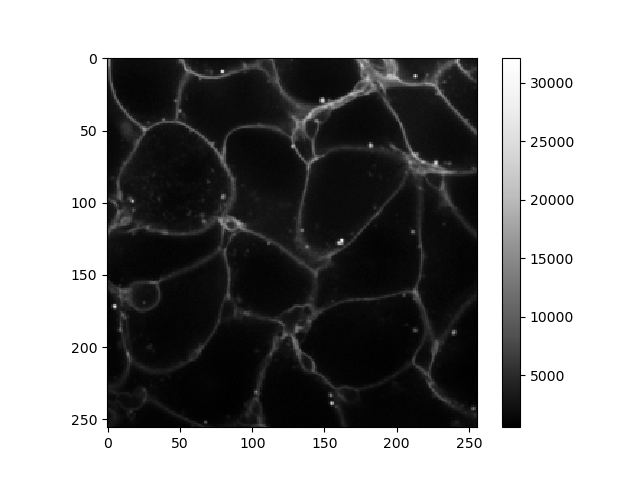cle._ image
 shape (256, 256) dtype float32 size 256.0 kB min 547.0 max 32145.0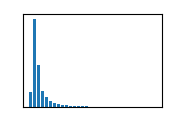This intensity gradient can be removed by dividing the image by its background, a Gaussian blurred version of it self.

intensity_equivalized = cle.divide_by_gaussian_background(image, sigma_x=10, sigma_y=10)
intensity_equivalized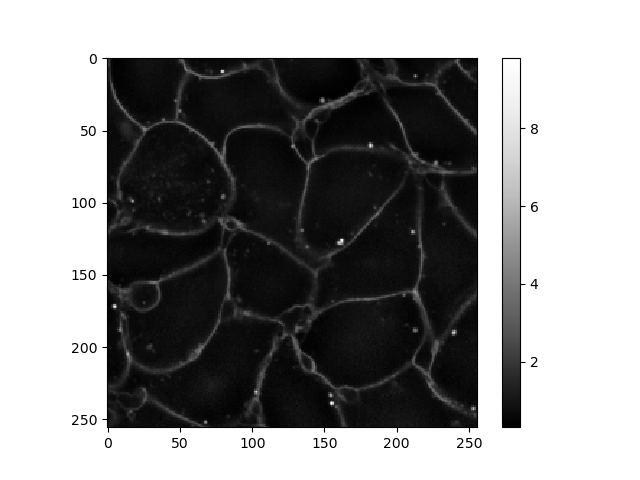cle._ image
 shape (256, 256) dtype float32 size 256.0 kB min 0.32106277 max 9.812795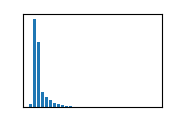## How it works#

To demonstrate how it works, we will do the same operation using two steps using scikit-image and numpy.

background = gaussian(image, sigma=10)
imshow(background, cmap="Greys_r")

C:\Users\haase\mambaforge\envs\bio39\lib\site-packages\skimage\io\_plugins\matplotlib_plugin.py:150: UserWarning: Low image data range; displaying image with stretched contrast.
lo, hi, cmap = _get_display_range(image)

<matplotlib.image.AxesImage at 0x1aa03625160>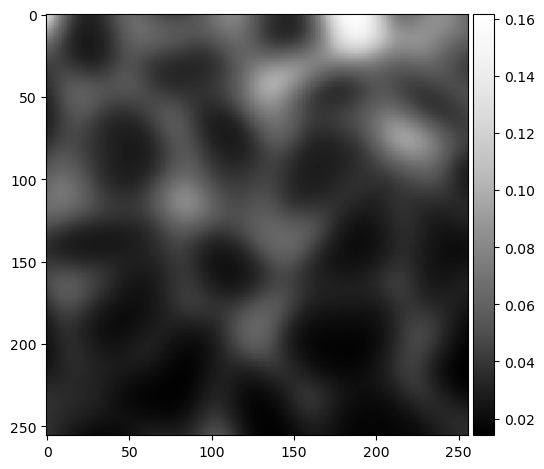result = image / background
imshow(result, cmap="Greys_r")

C:\Users\haase\mambaforge\envs\bio39\lib\site-packages\skimage\io\_plugins\matplotlib_plugin.py:150: UserWarning: Float image out of standard range; displaying image with stretched contrast.
lo, hi, cmap = _get_display_range(image)

<matplotlib.image.AxesImage at 0x1aa0350d580>